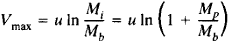# Rocket Equation

## Rocket Equation

the fundamental equation of the motion of a rocket. First published in 1903 by K. E. Tsiolkovskii in his article “Investigation of Interplanetary Space by Means of Rocket Devices,” it is called Tsiolkovskii’s formula in the Soviet literature.

The rocket equation gives the maximum velocity attained by a single-stage rocket in the ideal case—that is, when the flight occurs outside the earth’s atmosphere and gravitational field. The initial velocity of the rocket is assumed to be zero. The equation is often written in the formHere, Vmax is the maximum velocity attained by the rocket, that is, the velocity at burnout; u is the exhaust velocity; Mi is the total initial mass of the rocket; Mb is the mass remaining when burnout occurs; and Mp is the mass of the propellant that has been consumed. The quantity Mp/Mb is called the mass ratio (in Soviet usage, Tsiolkovskii number).

The rocket equation may be used for approximate estimates of the dynamic characteristics of the flight of a rocket when the drag and the force of gravity are small in comparison to the thrust developed by the rocket. Tsiolkovskii generalized the equation to the case of rocket motion in a uniform gravitational field.

The rocket equation gives only the upper limit of the velocity of a rocket. The actual velocity attained at burnout is always less than this upper limit because of the losses incurred in overcoming, for example, drag and the force of gravity during the rocket’s ascent. The rocket equation can be used to analyze the flight characteristics of multistage rockets.

A. A. KOSMODEM’IANSKII

References in periodicals archive ?
Due to the exponential nature of the rocket equation, intermediate tankings reduce the launch mass dramatically.
Launch vehicles are covered at the basic level of the rocket equation, the basic thermodynamical principles of rockets, and a basic mathematical introduction to staging calculations.
In the case of rocket propulsion, however, this term lies in the exponent of the all-hallowed rocket equation, so a doubling of [I.

Site: Follow: Share:
Open / Close